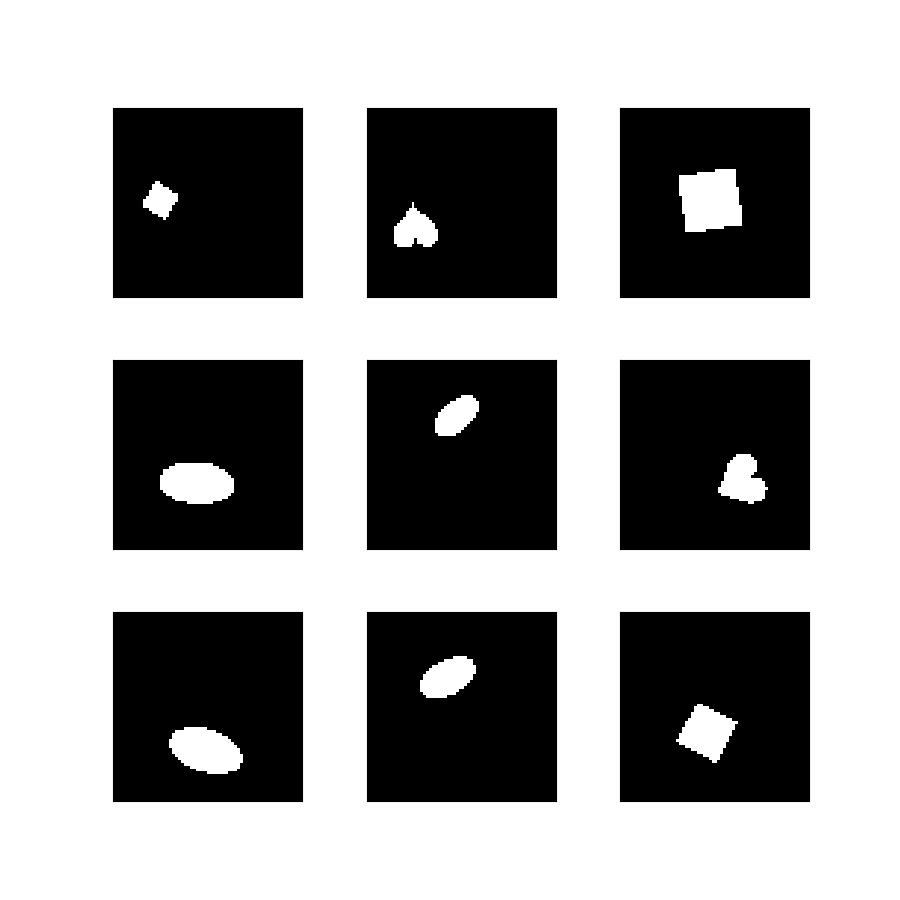# dsprites

• Description:

dSprites is a dataset of 2D shapes procedurally generated from 6 ground truth independent latent factors. These factors are color, shape, scale, rotation, x and y positions of a sprite.

All possible combinations of these latents are present exactly once, generating N = 737280 total images.

### Latent factor values

• Color: white
• Shape: square, ellipse, heart
• Scale: 6 values linearly spaced in [0.5, 1]
• Orientation: 40 values in [0, 2 pi]
• Position X: 32 values in [0, 1]
• Position Y: 32 values in [0, 1]

We varied one latent at a time (starting from Position Y, then Position X, etc), and sequentially stored the images in fixed order. Hence the order along the first dimension is fixed and allows you to map back to the value of the latents corresponding to that image.

We chose the latents values deliberately to have the smallest step changes while ensuring that all pixel outputs were different. No noise was added.

Split Examples
`'train'` 737,280
• Features:
``````FeaturesDict({
'image': Image(shape=(64, 64, 1), dtype=tf.uint8),
'label_orientation': ClassLabel(shape=(), dtype=tf.int64, num_classes=40),
'label_scale': ClassLabel(shape=(), dtype=tf.int64, num_classes=6),
'label_shape': ClassLabel(shape=(), dtype=tf.int64, num_classes=3),
'label_x_position': ClassLabel(shape=(), dtype=tf.int64, num_classes=32),
'label_y_position': ClassLabel(shape=(), dtype=tf.int64, num_classes=32),
'value_orientation': tf.float32,
'value_scale': tf.float32,
'value_shape': tf.float32,
'value_x_position': tf.float32,
'value_y_position': tf.float32,
})
``````
``````@misc{dsprites17,
author = {Loic Matthey and Irina Higgins and Demis Hassabis and Alexander Lerchner},
title = {dSprites: Disentanglement testing Sprites dataset},
howpublished= {https://github.com/deepmind/dsprites-dataset/},
year = "2017",
}
``````